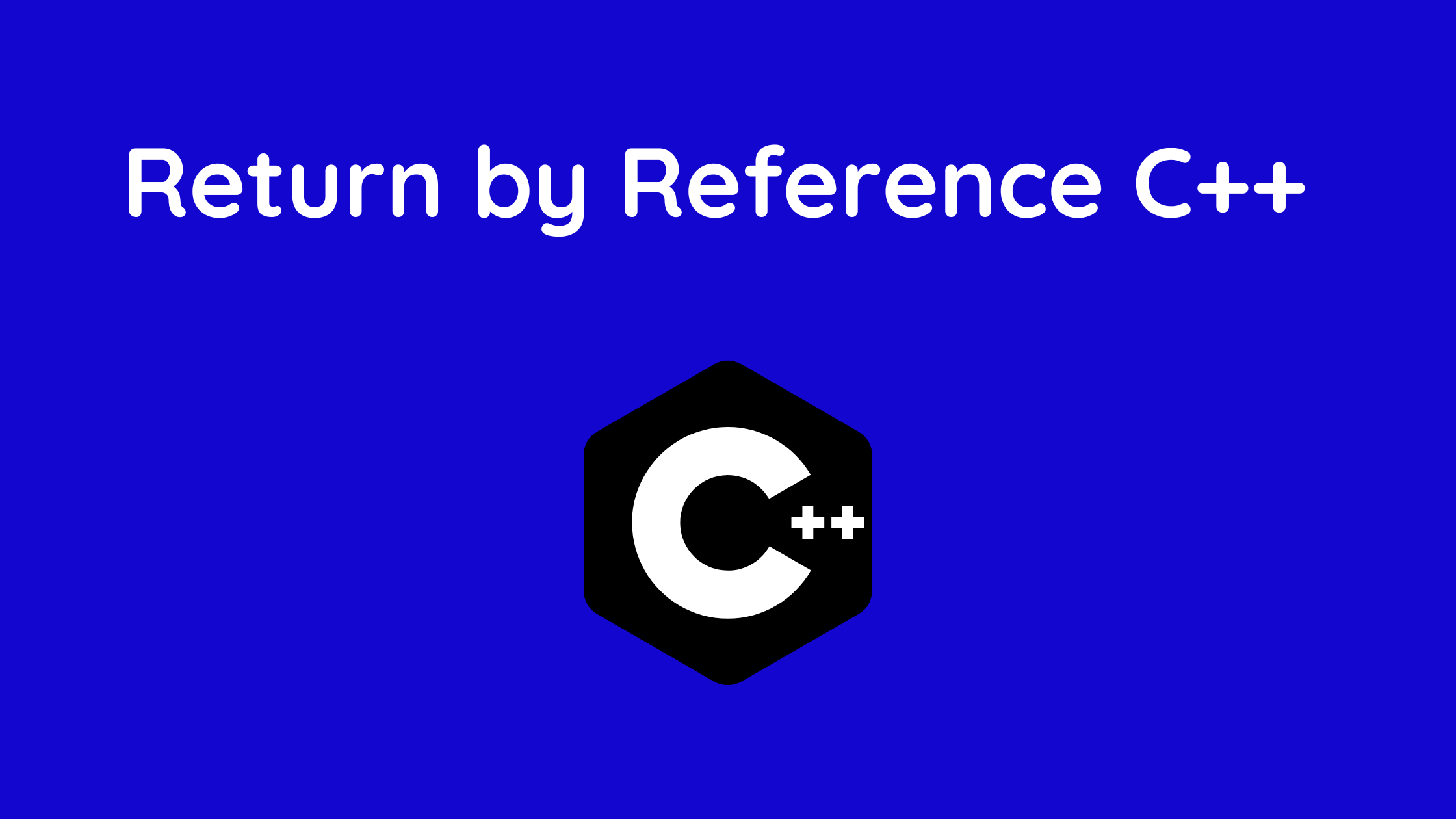## Return by Reference C++ with Example

You will discover how to effectively use a value return by reference to a function in this article.

In C++ programming, you can return a value by reference in addition to passing it by reference to a function.

To comprehend this functionality, you need to be familiar with the following:

Using references rather than pointers can make C++ programs easier to read and maintain. Similar to how it returns a pointer, a C++ function can also return a reference.

An implicit pointer to the return value is returned by a function when it returns a reference. A function may then be utilized on the left side of an assignment statement in this manner.

In C++, pointers and references were closely related to one another.

The main distinction is that while references are simply another variable’s alias, pointers can do operations like adding values.

In C++, functions can return a reference in addition to a pointer.
An implicit pointer is returned when a function returns a reference.

Similar to how it returns a pointer, a C++ function can also return a reference. Make that the item being referred to does not exit the scope while returning a reference.

Therefore, returning a reference to a local variable is prohibited. On the other hand, a static variable can always return a reference.
Call by reference and Returned by reference are extremely dissimilar.

When variables or pointers are returned as references, functions behave in a very fundamental way.

Global factors

Example:

```#include <iostream>
using namespace std;

// global variable
int num;

// function declaration
int& test();

int main() {

// assign 5 to num variable
// equivalent to num = 5;
test() = 5;

cout << num;

return 0;
}

// function definition
// returns the address of num variable
int& test() {
return num;
}
```

## Output

5

Test(return )’s type in the aforementioned application is int&. So, the variable num is referenced by this function.

Return num; is the return statement. In contrast, to return by value, this statement returns the variable itself rather than the value of num (address).

As a result, the variable can be given a value when it is returned, as in test() = 5;

This assigns the value 5 to the screen-displaying variable num.

## Things to Keep in Mind When Return by Reference

Unlike regular functions, which return values .this function does not. As a result, the function cannot return a constant.

int& test() {
return 2;
}

This function cannot return a local variable.

int& test() {
int n = 2;
return n;
}

You may like: